# Fano variety

A smooth complete irreducible algebraic varietyover a fieldwhose anti-canonical sheaf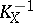is ample (cf. Ample sheaf). The basic research into such varieties was done by G. Fano (, ).
A Fano variety of dimension 2 is called a del Pezzo surface and is a rational surface. The multi-dimensional analogues of del Pezzo surfaces — Fano varieties of dimension— are not all rational varieties, for example the general cubic in the projective space. It is not known (1984) whether all Fano varieties are unirational.
The Picard group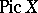of a three-dimensional Fano variety is finitely generated and torsion-free. In case the ground fieldis, the rank of, which is equal to the second Betti number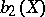, does not exceed 10 (see ). If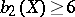, then the Fano variety is isomorphic to, where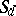is the del Pezzo surface of order. A Fano varietyis called primitive if there is no monoidal transformation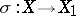to a smooth varietywith centre at a non-singular irreducible curve. Ifis a primitive Fano variety, then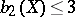. If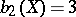, thenis a conic fibre space over, in other words, then there is a morphismeach fibre of which is isomorphic to a conic, that is, an algebraic scheme given by a homogeneous equation of degree 2 in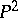. A Fano varietywith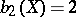is a conic fibre space over the projective plane(see ). In the case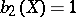there are 18 types of Fano varieties, which have been classified (see ).
For three-dimensional Fano varietiesthe self-intersection index of the anti-canonical divisor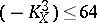. The largest integer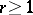such that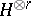is isomorphic tofor some divisor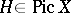is called the index of the Fano variety. The index of a three-dimensional Fano variety can take the values 1, 2, 3, or 4. A Fano variety of index 4 is isomorphic to the projective space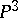, and a Fano variety of index 3 is isomorphic to a smooth quadric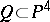. If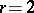, then the self-intersection indexcan take the values, with each of them being realized for some Fano variety. For a Fano variety of index 1 the mapping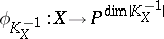defined by the linear system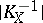has degree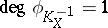or 2. The Fano varieties of index 1 for whichhave been classified. If, thencan be realized as a subvarietyof degree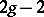in the projective space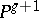. The numberis called the genus of the Fano variety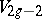and is the same as the genus of the canonical curve — the section ofunder the anti-canonical imbedding into. The Fano varietiesthe class of a hyperplane section of which is the same as the anti-canonical class and generateshave been classified (see , ).